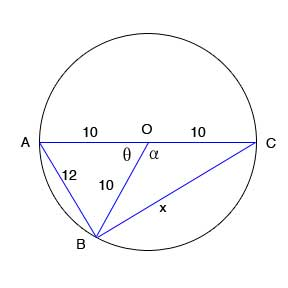SEARCH HOMEMath Central Quandaries & QueriesBeary, a student: AOC is a diameter of circle O. Line AB is 12, and lines OA and OC (the radii) are 10. Find the length of line BO and chord BC.Hi Beary,

Remember that if O is the centre then OB will also be a radius of 10.You first need to solve for angle θ using the law of cosine states:
For any triangle with side lengths a, b and c,
c2 = a2 + b2 - 2ab cos C
where angle C is the angle opposite side length c.
In your case, side AB is opposite to θ.

Next solve for α which we know equals 180- θ since it makes a straight angle with θ. Use the value of α and the law of cosines again to solve for side BC.

Hope this helps,

JaniceMath Central is supported by the University of Regina and The Pacific Institute for the Mathematical Sciences.